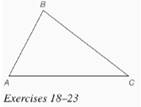Chapter 7.CR, Problem 19CRElementary Geometry For College St...

7th Edition
Alexander + 2 others
ISBN: 9781337614085

Solutions

Chapter
SectionElementary Geometry For College St...

7th Edition
Alexander + 2 others
ISBN: 9781337614085
Textbook Problem

In Review Exercises 18 to 23, use construction methods with the accompanying figure. Given: Δ A B CFind: The circumcenter

To determine

To find: The circumcenter of the triangle ABC.

Explanation

Given:

The triangle ABC is shown below.

Definition used:

Circumcenter:

The point at which the perpendicular bisectors of the sides of a triangle meet is the circumcenter of the triangle.

Calculation:

Consider the triangle ABC.

Draw the perpndicular bisectors for the sides AB, BC, CA of the triangle ABC.

Still sussing out bartleby?

Check out a sample textbook solution.

See a sample solution

The Solution to Your Study Problems

Bartleby provides explanations to thousands of textbook problems written by our experts, many with advanced degrees!

Get Started

In Problems 19-44, factor completely. 25.

Mathematical Applications for the Management, Life, and Social Sciences

Sketch the graphs of the equations in Exercises 512. xy=2x3+1

Finite Mathematics and Applied Calculus (MindTap Course List)

Evaluate the integral. 31. tan5xdx

Single Variable Calculus: Early Transcendentals

The solution to y = y2 with y(1)=13 is: a) y=1x+2 b) y=lnx+13 c) y=12x216 d) y=12x16

Study Guide for Stewart's Single Variable Calculus: Early Transcendentals, 8th

True or False: , for –π ≤ t ≤ π, is smooth.

Study Guide for Stewart's Multivariable Calculus, 8th